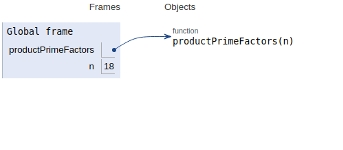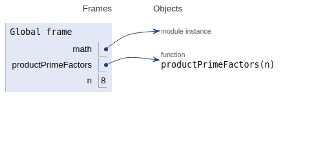# Product of unique prime factors of a number in Python Program

PythonServer Side ProgrammingProgramming

## Problem statement

Given a number n, we need to find the product of all of its unique prime factors available and return it.

### For Example

Input: num = 11
Output: Product is 11

### Explanation

Here, the input number is 11 having only 1 prime factor and it is 11. And hence their product is 11.

## Approach 1

Using a for loop from i = 2 to n+1 check whether i is a factor of n & then check if i is the prime number itself, if yes then store product in product variable and continue this process until till i becomes = n.

## Example

Live Demo

def productPrimeFactors(n):
product = 1
for i in range(2, n+1):
if (n % i == 0):
isPrime = 1
for j in range(2, int(i/2 + 1)):
if (i % j == 0):
isPrime = 0
break
if (isPrime):
product = product * i
return product
# main
n = 18
print (productPrimeFactors(n))

## Output

120

The scope of all the variables are shown in the image below −## Approach 2

1) While n is divisible by 2(even), print 2 and divide n by 2.

2) After step 1, n must become odd. Now start a for loop from i = 3 till the square root of n. While i divides n, print i and divide n by i. After i fails to divide n, increment i by 2 and continue the process.

3) If n is a prime number and is greater than 2, then n will not become 1 by above two steps. Hence print n if it is greater than 2.

## Example

Live Demo

import math
def productPrimeFactors(n):
product = 1
# prime factor 2
if (n % 2 == 0):
product *= 2
while (n%2 == 0):
n = n/2
# n must be odd
for i in range (3, int(math.sqrt(n)), 2):
# While i divides n, print i and
# divide n
if (n % i == 0):
product = product * i
while (n%i == 0):
n = n/i
# n is a prime number greater than 2
if (n > 2):
product = product * n
return product
# main()
n = 8
print (int(productPrimeFactors(n)))

## Output

2

The scopes of the variables are mentioned in the image below −## Conclusion

In this article, we learned about the product of unique prime factors of a given number with a brute force approach and an efficient approach